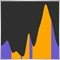# How to Calculate Profit/Loss percentage change48

Hello, does anyone have a formula to calculate the change percentage as shown below? thanks

Files:
Capture.PNG 22 kb3621

mrwick:

Hello, does anyone have a formula to calculate the change percentage as shown below? thanks

(CurrentPrice - OpenPrice) / OpenPrice * 10048

Ehsan Tarakemeh:
(CurrentPrice - OpenPrice) / OpenPrice * 100
`DoubleToString((((SymbolInfoDouble(_Symbol,SYMBOL_BID)-PositionGetDouble(POSITION_PRICE_OPEN)) / PositionGetDouble(POSITION_PRICE_OPEN)) *100), 2)`

Thanks. Sample code above for anyone looking for this.